Quadratic equations can be solved by factorising, completing the square and using a formula. In this section you will learn how to:

• solve quadratic equations by factorising
• solve quadratic equations by completing the square
• solve quadratic equations by using the formula
• solve simultaneous equations when one of them is quadratic

This animated video states that a quadratic is an expression featuring an unknown number which has been squared. Examples are used to show how to simplify quadratics by factorisation. Working with both positive and negative terms is shown. Plotting a quadratic equation on a graph is used to show why quadratics can have more than one value.

Unless a graphical method is asked for, quadratic equations on the non-calculator paper will probably involve factorising or completion of the square. Quadratic equations can have two different solutions or roots.

You may need a quick look at 'factorising' again to remind yourself how to factorise expressions such as:
x2 − x − 6
which factorises into (x − 3)(x + 2),

a2 − 3a
which factorises into a(a − 3)
and

b2 − 2b + 1
which will factorise into (b − 1)2.

This video shows you how to solve a quadratic equation by factoring.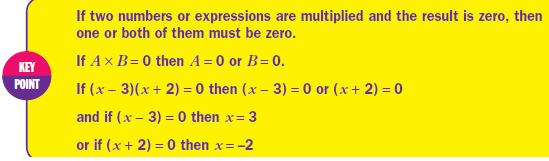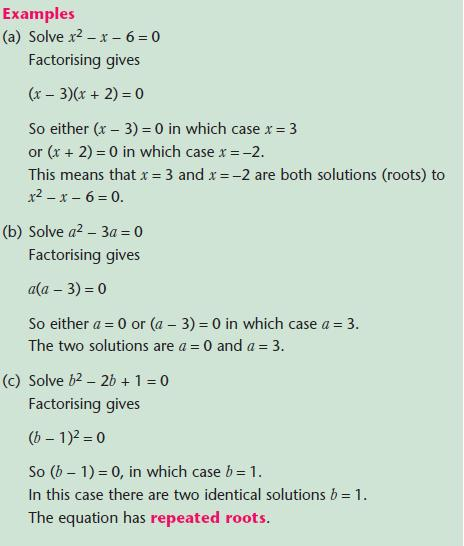Solving quadratic equations by completing the square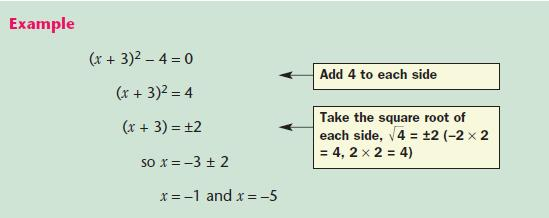NOTE: Check by substituting both roots back into the original equation.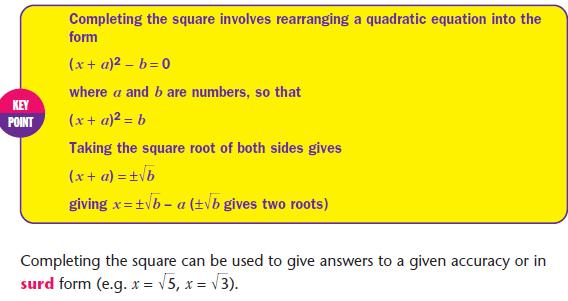This following is a common way to lead into asking you to use completion of the square.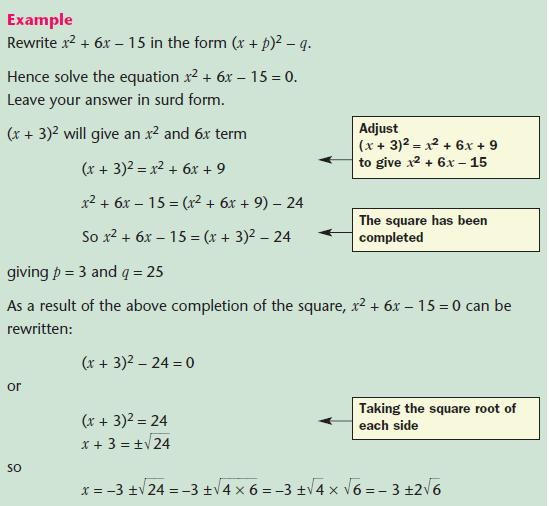NOTE: Remember in, for example, (x + n)2 the number of xs (called the coefficient of x) is 2n. So the coefficient of x will be 6 in (x + 3)2.

This video shows an animated guide to simplifying quadratic expressions and equations by completing the square. Examples are shown of how to complete the square to factorise any expression and to solve equations.

Solving quadratic equations by using the formula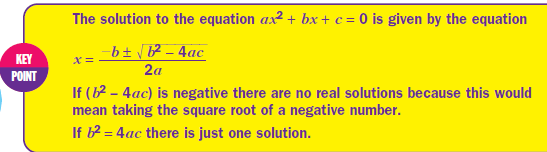When using the quadratic formula, don’t forget the ‘2a’denominator. Also, be careful when dealing with negative numbers
inside the square root. State your values of a,b and c to be used in the formula.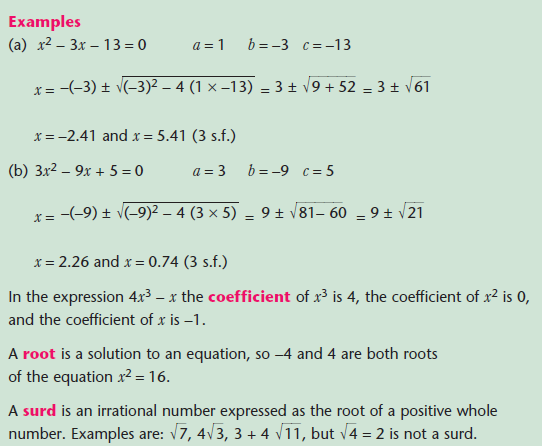NOTE: The above calculations are easily checked − especially if your calculator can store numbers as variables.